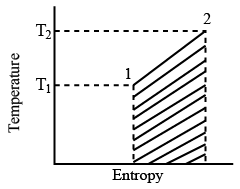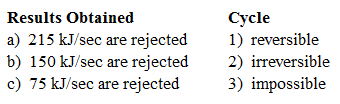# ISRO Scientist or Engineer Mechanical 2007

Instructions

For the following questions answer them individually

Question 1

# Only rocket engines can be propelled to space becauseQuestion 2

# The universal gas constant of a gas is the product of molecular weight of the gas andQuestion 3

# Vander Waal’s equation of state of a gas isQuestion 4

# The radiative heat transfer rate per unit area (W/m$$^2$$) between two plane parallel grey surfaces (emissivity = 0.9) maintained at 400 K and 300 K is (Stefan Bottzman constant$$\sigma = 5.67 \times 10^{-8} W/m^2 K^4$$)Question 5

# The efficiency $$\eta$$ of any heat engine and efficiency $$\eta_R$$ of a reversible heat engine operating between common heat source and heat sink are related asQuestion 6

# A spherical shaped vessel of 1.4 m outer diameter is 90 mm thick. Find the rate of heat leakage, if the temperature difference between the inner and outer surfaces is $$220^\circ C$$. Thermal conductivity of the material of the sphere is 0.083 W/m K.Question 7

# The velocity componentsin the x and y directions are given by $$u = \lambda xy^3 - x^2y; v = xy^2 - \frac{3}{4}y^4$$ The value of $$\lambda$$ for a possible flow field involving an incompressible fluid isQuestion 8

# The temperature field in a body varies according to the equation $$T(x, y) = x^3 + 4xy$$. The direction cf fastest variation in temperature at the point (1, 0) is given byQuestion 9

# The temperature distribution at a certain input of time in concrete slab during curing is given by $$T = 3x^2 + 3x + 16$$ where x is in cm and T is in K. The rate of change of temperature with time is given ($$\alpha = 0.0003 cm^2/sec$$)Question 10

# 300 kJ/sec of heat is applied at a constant fixed temperature of $$290^\circ C$$ to a heat engine. The heat rejection takes place at $$8.5^\circ C$$. then match the following:OR